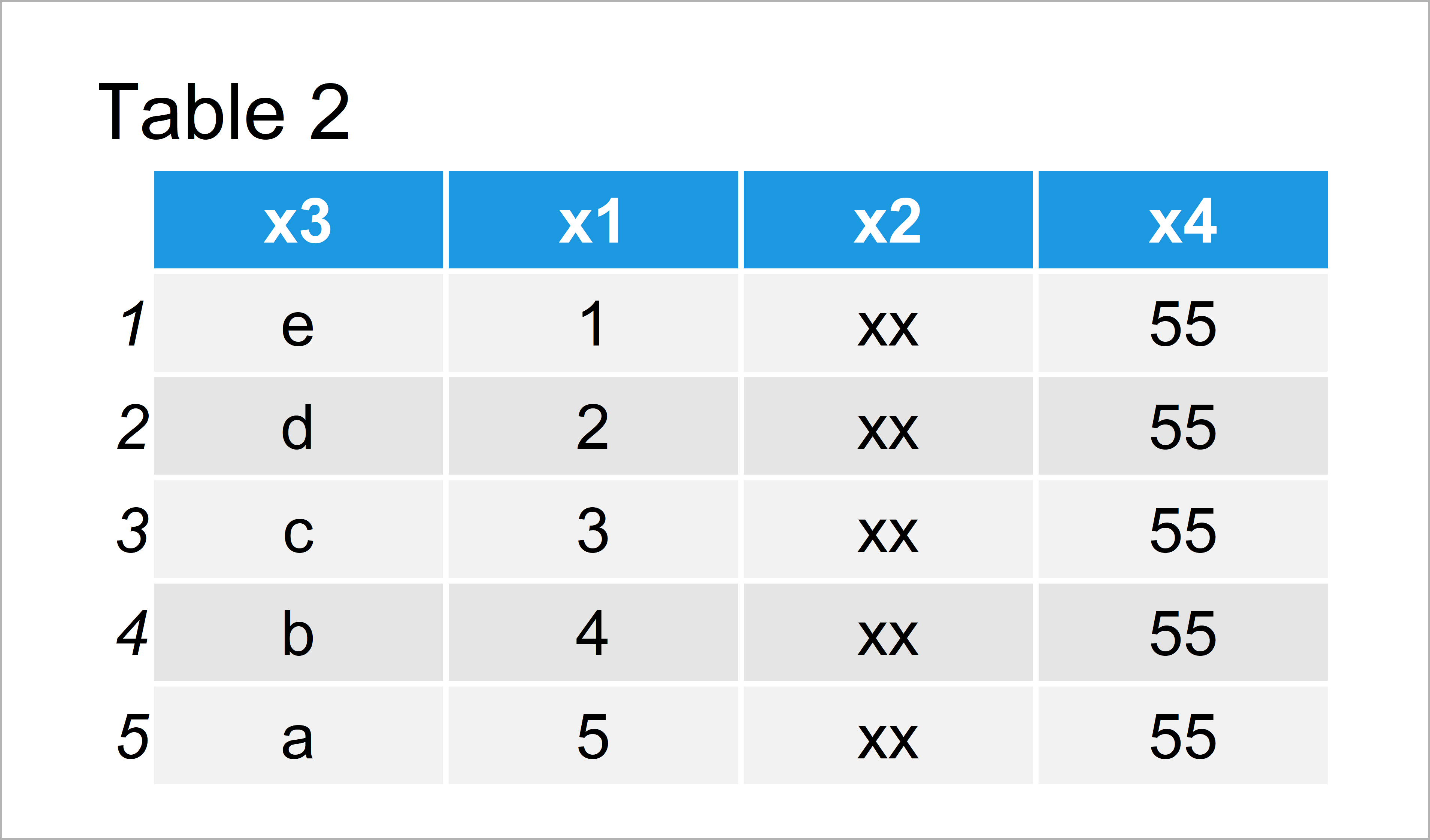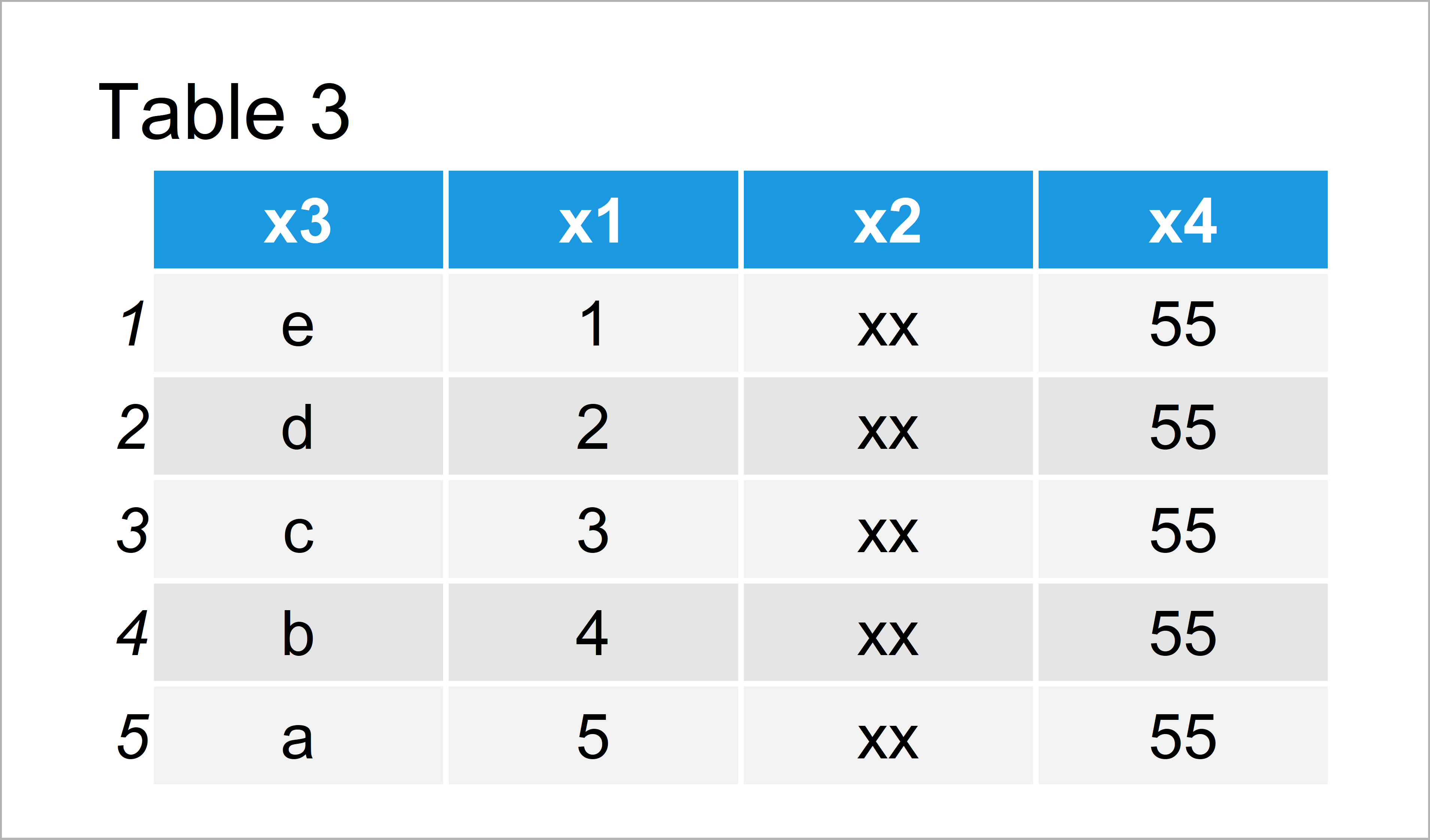# Move Column to First Position of Data Frame in R (2 Examples)

In this R programming tutorial you’ll learn how to shift a variable to the beginning of a data frame.

The tutorial consists of these contents:

So now the part you have been waiting for – the exemplifying R code:

## Creating Example Data

Let’s first construct some example data in R:

```data <- data.frame(x1 = 1:5,    # Create data frame
x2 = "xx",
x3 = letters[5:1],
x4 = 55)
data                            # Print data frame```As you can see based on Table 1, our example data is a data frame made of five rows and four columns.

## Example 1: Shift Column to First Position of Data Frame Using Base R

In Example 1, I’ll show how to move a data frame column at the beginning of the data frame using the basic installation of the R programming language.

For this, we can use the c() and names functions in combination with the != operator:

```data_new1 <- data[ , c("x3",    # Reorder data frame
names(data)[names(data) != "x3"])]
data_new1                       # Print updated data frame```The output of the previous R programming syntax is shown in Table 2: As you can see, we have moved the variable x3 to the beginning of the data frame.

## Example 2: Shift Column to First Position of Data Frame Using dplyr Package

The following R syntax illustrates how to use the functions of the dplyr environment to set the position of a variable to the beginning of a data frame.

We first have to install and load the dplyr package:

```install.packages("dplyr")       # Install dplyr package

Next, we can use the select and everything functions to move a variable from the middle of our data frame to the first index position as shown below:

```data_new2 <- data %>%           # Reorder data frame
dplyr::select("x3", everything())
data_new2                       # Print updated data frame```As shown in Table 3, we have created a reordered version of our input data frame where the variable x3 was set to be at the first position.

## Video & Further Resources

In case you need further information on the R programming codes of the present article, you may have a look at the following video which I have published on my YouTube channel. I illustrate the R syntax of this article in the video.

Please accept YouTube cookies to play this video. By accepting you will be accessing content from YouTube, a service provided by an external third party.If you accept this notice, your choice will be saved and the page will refresh.

Furthermore, you could read the other articles of this website. Some other tutorials on related topics such as vectors, missing data, merging, and lists can be found below.

Summary: You have learned in this tutorial how to move data frame columns to different positions in the R programming language. In case you have any further questions, don’t hesitate to let me know in the comments below.

Subscribe to the Statistics Globe Newsletter